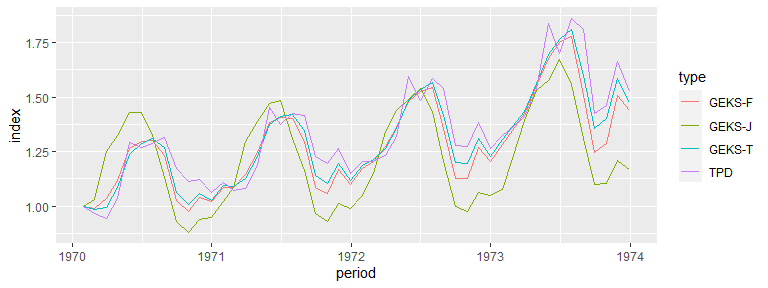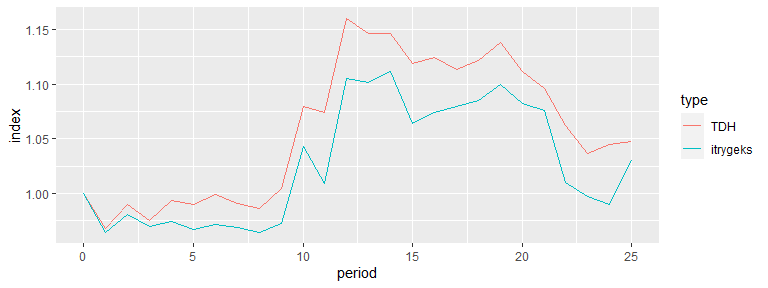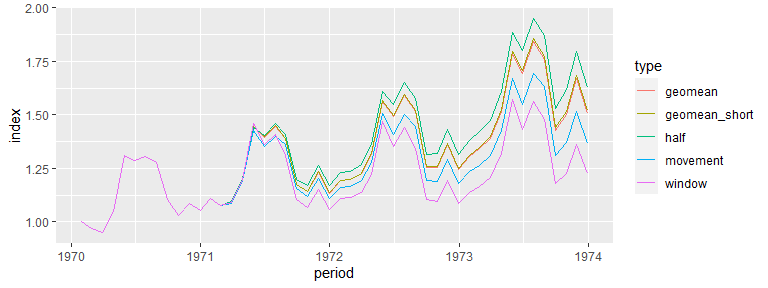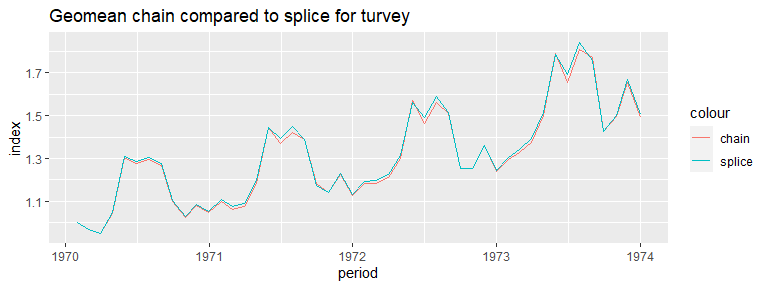# Multilateral

## Overview

The multilateral package provides one user facing function, that is multilateral(). The user provides the necessary attributes of the dataset for the respective multilateral method.

This returns a list object containing the continuous spliced index, each individual windows calculation (useful for diagnostics), and splicing information if applicable.

#devtools::install_github("MjStansfi/multilateral")
library(multilateral)

tpd_index <- multilateral(period = turvey$month, id = turvey$commodity,
price = turvey$price, quantity = turvey$quantity,
splice_method = "geomean",
window_length = 13,
index_method = "TPD")
str(tpd_index)
#> List of 3
#>  $index :Classes 'data.table' and 'data.frame': 48 obs. of 3 variables: #> ..$ period   : Date[1:48], format: "1970-01-31" "1970-02-28" ...
#>   ..$index : num [1:48] 1 0.971 0.949 1.047 1.308 ... #> ..$ window_id: int [1:48] 1 1 1 1 1 1 1 1 1 1 ...
#>   ..- attr(*, ".internal.selfref")=<externalptr>
#>  $index_windows:Classes 'data.table' and 'data.frame': 468 obs. of 3 variables: #> ..$ period   : Date[1:468], format: "1970-01-31" "1970-02-28" ...
#>   ..$index : num [1:468] 1 0.971 0.949 1.047 1.308 ... #> ..$ window_id: int [1:468] 1 1 1 1 1 1 1 1 1 1 ...
#>   ..- attr(*, ".internal.selfref")=<externalptr>
#>  $splice_detail:Classes 'data.table' and 'data.frame': 35 obs. of 5 variables: #> ..$ period                : Date[1:35], format: "1971-02-28" "1971-03-31" ...
#>   ..$latest_window_movement: num [1:35] 0.97 1.012 1.097 1.195 0.949 ... #> ..$ revision_factor       : num [1:35] 1 1 1 1.01 1.02 ...
#>   ..$update_factor : num [1:35] 0.972 1.013 1.099 1.205 0.966 ... #> ..$ window_id             : int [1:35] 2 3 4 5 6 7 8 9 10 11 ...
#>   ..- attr(*, ".internal.selfref")=<externalptr>
#>  - attr(*, "class")= chr [1:2] "list" "multilateral"
#>  - attr(*, "params")=List of 6
#>   ..$index_method : chr "TPD" #> ..$ window_length   : num 13
#>   ..$splice_method : chr "geomean" #> ..$ chain_method    : NULL
#>   ..$check_inputs_ind: logi TRUE #> ..$ matched         : NULL

## Show case of index methods you can apply

The package provides two lazily loaded datasets, they are ‘turvey’ and ‘synthetic_gfk’. The first dataset provides price and quantity information for products with a unique ID. The second dataset provides price and quantity information for products with a unique ID, however it also includes de-identified characteristics.

Note for this example we do not provide a window length, therefore the index is calculated over the whole dataset.

str(turvey)
#> 'data.frame':    176 obs. of  4 variables:
#>  $month : Date, format: "1970-01-31" "1970-01-31" ... #>$ commodity: Factor w/ 5 levels "Apples","Grapes",..: 1 2 3 1 2 3 1 2 3 1 ...
#>  $price : num 1.14 2.48 1.3 1.17 2.75 1.25 1.17 5.07 1.21 1.4 ... #>$ quantity : int  3086 82 10266 3765 35 9656 4363 9 7940 4842 ...
library(ggplot2)

index_methods <- c("TPD","GEKS-T","GEKS-J","GEKS-F")

start_run_time <- Sys.time()
turvey_multilats <- lapply(index_methods, function(index_method){

temp_index <- multilateral(period = turvey$month, id = turvey$commodity,
price = turvey$price, quantity = turvey$quantity,
index_method = index_method)

#For identification in plot
temp_index$index$type <- index_method

return(temp_index$index) }) end_run_time <- Sys.time() turvey_multilats <- do.call(rbind,turvey_multilats) plot <- ggplot(turvey_multilats)+geom_line(aes(x = period, y = index, colour = type)) print(plot)Below indicates the time taken to run all four index methods above, highlighting efficiency of calculation print(end_run_time-start_run_time) #> Time difference of 8.334987 secs ### Data sources with features The above methods could also be applied to this dataset str(synthetic_gfk) #> 'data.frame': 5509 obs. of 15 variables: #>$ month_num : int  0 1 2 3 4 5 6 7 8 9 ...
#>  $char11 : Factor w/ 10 levels "brand_a","brand_b",..: 1 1 1 1 1 1 1 1 1 1 ... #>$ char1     : num  10.6 10.6 10.6 10.6 10.6 10.6 10.6 10.6 10.6 10.6 ...
#>  $char2 : int 16006 16006 16006 16006 16006 16006 16006 16006 16006 16006 ... #>$ char3     : Factor w/ 19 levels "val_a","val_b",..: 18 18 18 18 18 18 18 18 18 18 ...
#>  $char4 : Factor w/ 4 levels "val_a","val_b",..: 1 1 1 1 1 1 1 1 1 1 ... #>$ char5     : Factor w/ 5 levels "val_a","val_b",..: 1 1 1 1 1 1 1 1 1 1 ...
#>  $char6 : Factor w/ 88 levels "FBOK6552","GR3000",..: 49 49 49 49 49 49 49 49 49 49 ... #>$ char7     : Factor w/ 3 levels "AAA","BBB","CCC": 3 3 3 3 3 3 3 3 3 3 ...
#>  $char8 : Factor w/ 2 levels "100M","150D": 2 2 2 2 2 2 2 2 2 2 ... #>$ char9     : Factor w/ 4 levels "A100","A450",..: 3 3 3 3 3 3 3 3 3 3 ...
#>  $char10 : Factor w/ 5 levels "abb","bbb","bhy",..: 5 5 5 5 5 5 5 5 5 5 ... #>$ prodid_num: int  3 3 3 3 3 3 3 3 3 3 ...
#>  $quantity : int 280 126 148 56 69 43 22 21 9 17 ... #>$ value     : int  196420 85312 95920 38552 47397 28303 14812 13701 6304 10651 ...

Now we wrangle this into the necessary format, that is a unique observation for any given period.

library(dplyr)

synthetic_gfk <- synthetic_gfk%>%
group_by(month_num,prodid_num)%>%
mutate(quantity = sum(quantity),
value = sum(value))%>%
ungroup()%>%
unique

#Calculate the unit value (price)
synthetic_gfk$uv <- synthetic_gfk$value/synthetic_gfk$quantity #Extract data.frame containing features of interest features <-synthetic_gfk[,grepl("char",colnames(synthetic_gfk))] Once the dataframe is in the correct format we can run it through the main function with index_method set to ‘GEKS-IT’ and provide a features argument, containing the dataframe of features. Note GEKS-IT still requires a product id.  itrygeks_index <- multilateral(period = synthetic_gfk$month_num,
id = synthetic_gfk$prodid_num, price = synthetic_gfk$uv,
quantity = synthetic_gfk$quantity, features = features, splice_method = "geomean", index_method = "GEKS-IT") #For identification in plot itrygeks_index$index$type <- "itrygeks" Alternatively we could run a basic TDH model, which does not require a unique ID.  #Note no unique product ID TDH_index <- multilateral(period = synthetic_gfk$month_num,
price = synthetic_gfk$uv, quantity = synthetic_gfk$quantity,
features = features,
splice_method = "geomean",
index_method = "TDH")

#For identification in plot
TDH_index$index$type <- "TDH"
feature_indexes <- rbind(itrygeks_index$index,TDH_index$index)

plot <- ggplot(feature_indexes)+geom_line(aes(x = period, y = index, colour = type))

print(plot)## Show case of splice methods you can apply

Here we introduce a window length and therefore some way to chain together the indexes. To do this and still meet the no revision constrant we ‘splice’ the individual windows together.


splice_methods <- c("geomean","geomean_short","window","movement","half")
turvey_splices <- lapply(splice_methods, function(splice_method){

temp_index <- multilateral(period = turvey$month, price = turvey$price,
id = turvey$commodity, quantity = turvey$quantity,
window_length = 13,
splice_method = splice_method,
index_method = "TPD")

temp_index$index$type <- splice_method

return(temp_index$index) }) turvey_splices <- do.call(rbind,turvey_splices) plot <- ggplot(turvey_splices)+geom_line(aes(x = period, y = index, colour = type)) print(plot)## Retrospective splicing (chained indexes) Typical splicing of a multilateral index exists to enforce the no revision constraint for which most official price indexes follow. However this package allows for the user to provide a ‘chain_method’ rather than a ‘splice_method’. With this we are able to look at what the index would be if we used all information available as at the latest period. Note: depending on the position of the chaining, the series will be a different length.  chain_methods <- c("geomean","window","movement","half") turvey_chains <- lapply(chain_methods, function(chain_method){ temp_index <- multilateral(period = turvey$month,
price = turvey$price, id = turvey$commodity,
quantity = turvey$quantity, window_length = 13, # splice_method = splice_method, chain_method = chain_method, index_method = "TPD") temp_index$index$type <- chain_method return(temp_index$index)
})

turvey_chains <- do.call(rbind,turvey_chains)

plot <- ggplot()+
geom_line(aes(x = period, y = index, colour = "chain"), data = turvey_chains[turvey_chains$type=="geomean"])+ geom_line(aes(x = period, y = index, colour = "splice"), data = turvey_splices[turvey_splices$type=="geomean"])+
ggtitle("Geomean chain compared to splice for turvey")

print(plot)## Why this package

• This package is fast relative to other available packages
• This package allows flexibility of user input
• This package has the flexibility to incorporate new methods if needed
• The methods available are those that Stats NZ are either using or intending to explore the use of
• Building own package brings transparency and understanding to methods and nature of the functions

Below we compare the PriceIndices, IndexNumR, and multilateral package. We look at how you would calculate the same index.

library(multilateral)
library(IndexNumR)
library(PriceIndices)
library(dplyr)
#---------------------#multilateral package
turvey_multilateral <- multilateral(period = turvey$month, price = turvey$price,
quantity = turvey$quantity, id = turvey$commodity,
window_length = 13,
splice_method = "geomean",
index_method = "GEKS-F",
check_inputs_ind = T)

#---------------------#PriceIndices package

#Requires data.frame with specific colnames, and format of time

turvey_mod <- turvey%>%select(time = month,
prodID = commodity,
prices = price,
quantities = quantity)

turvey_priceindices <- geks_splice(turvey_mod, "1970-01", "1973-12", 13, splice = "mean", interval = T)

#---------------------#IndexNumR package

#Requires time to be a sequential numeric vector

turvey <- turvey%>%mutate(month_num = as.numeric(as.factor(month)))

turvey_IndexNumR <- GEKSIndex(turvey,
pvar = "price",
qvar = "quantity",
pervar = "month_num",
prodID = "commodity",
indexMethod = "fisher",
window = 13,
splice = "mean")

Note the multilateral function also has a ‘num_cores’ parameter for parallelisation. Because calculation over a given window is not dependent on another, the majority of the function computation time - calculating indexes over each window - can be done in parallel. This is only applicable when there is more than one window, and only becomes noticeably quicker when dealing with larger datasets (>500k rows) as distributing to multiple cores has overheads. It is up to the user to find the correct balance.

nrow(big_data)
# 100000000

multilateral(period = big_data$month, price = big_data$price,
quantity = big_data$quantity, id = big_data$commodity,
window_length = 13,
splice_method = "geomean",
index_method = "TPD",
check_inputs_ind = T,
num_cores = 32)